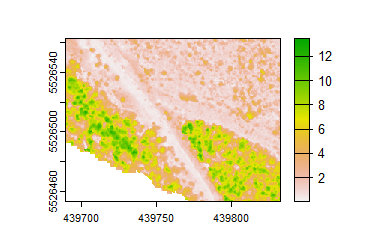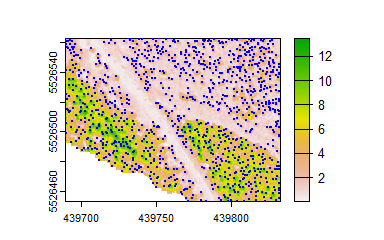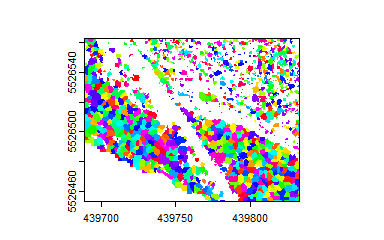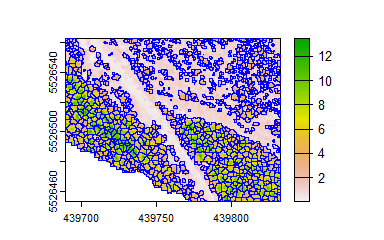# Canopy Analysis in R Using ForestTools

library(ForestTools)

This short guide will demonstrate how to use the ForestTools package to detect and outline trees from a canopy height model (CHM) derived from a photogrammetric point cloud.

Begin by installing ForestTools.

install.packages("ForestTools")

A sample CHM is included in the ForestTools package. It represents a small 1.5 hectare swath of forest in the Kootenay Mountains, British Columbia.

# Attach the 'ForestTools' and 'terra' libraries
library(ForestTools)
library(terra)
library(sf)

# Load sample canopy height model
chm <- terra::rast(kootenayCHM)

View the CHM using the plot function. The cell values are equal to the canopy’s height above ground.

# Remove plot margins (optional)
par(mar = rep(0.5, 4))

# Plot CHM (extra optional arguments remove labels and tick marks from the plot)
plot(chm, xlab = "", ylab = "", xaxt='n', yaxt = 'n')# Detecting treetops

Dominant treetops can be detected using vwf. This function implements the variable window filter algorithm developed by Popescu and Wynne (2004). In short, a moving window scans the CHM and tags a given cell as treetop if it is found to be the highest within the window. The size of the window itself changes dynamically depending on the height of the cell on which it is centered. This is to account for varying crown sizes, with tall trees assumed to have wide crowns and vice versa.

Therefore, the first step is to define the function that will define the dynamic window size. Essentially, this function should take a CHM cell value (i.e.: the height of the canopy above ground at that location) and return the radius of the search window. Here, we will define a simple linear equation, but any function with a single input and output will work. We do not wish for the vwf to tag low-lying underbrush or other spurious treetops, and so we also set a minimum height of 2 m using the minHeight argument. Any cell with a lower value will not be tagged as a treetop.

# Function for defining dynamic window size
lin <- function(x){x * 0.05 + 0.6}

# Detect treetops
ttops <- vwf(chm, winFun = lin, minHeight = 2)

We can now plot these treetops on top of the CHM.

# Plot CHM
plot(chm, xlab = "", ylab = "", xaxt='n', yaxt = 'n')

# Add dominant treetops to the plot
plot(ttops$geometry, col = "blue", pch = 20, cex = 0.5, add = TRUE)The ttops object created by vwf in this example contains the spatial coordinates of each detected treetop, as well as two default attributes: height and winRadius. These correspond to the tree’s height above ground and the radius of the moving window where the tree was located. Note that winRadius is not necessarily equivalent to the tree’s crown radius. # Get the mean treetop height mean(ttops$height)
##  5.404217

# Outlining tree crowns

Canopy height models often represent continuous, dense forests, where tree crowns abut against each other. Outlining discrete crown shapes from this type of forest is often referred to as canopy segmentation, where each crown outline is represented by a segment. Once a set of treetops have been detected from a canopy height model, the mcws function can be used for this purpose.

The mcws function implements the watershed algorithm from the imager library. Watershed algorithms are frequently used in topographical analysis to outline drainage basins. Given the morphological similarity between an inverted canopy and a terrain model, this same process can be used to outline tree crowns. However, a potential problem is the issue of oversegmentation, whereby branches, bumps and other spurious treetops are given their own segments. This source of error can be mitigated by using a variant of the algorithm known as marker-controlled watershed segmentation (Beucher & Meyer, 1993), whereby the watershed algorithm is constrained by a set of markers – in this case, treetops.

The mcws function also takes a minHeight argument, although this value should be lower than that which was assigned to vwf. For the latter, minHeight defines the lowest expected treetop, whereas for the former it should correspond to the height above ground of the fringes of the lowest trees.

# Create crown map
crowns_ras <- mcws(treetops = ttops, CHM = chm, minHeight = 1.5)

# Plot crowns
plot(crowns_ras, col = sample(rainbow(50), nrow(unique(chm)), replace = TRUE), legend = FALSE, xlab = "", ylab = "", xaxt='n', yaxt = 'n')By default, mcws returns a raster, where each crown is given a unique cell value. Depending on the intended purpose of the crown map, it may be preferable to store these outlines as polygons. This can be accomplished by setting the format argument to “polygons”. As an added benefit, these polygons will inherit the attributes of the treetops from which they were generated, such as height.

# Create polygon crown map
crowns_poly <- mcws(treetops = ttops, CHM = chm, format = "polygons", minHeight = 1.5)

# Plot CHM
plot(chm, xlab = "", ylab = "", xaxt='n', yaxt = 'n')

# Add crown outlines to the plot
plot(crowns_poly$geometry, border = "blue", lwd = 0.5, add = TRUE)Assuming that each crown has a roughly circular shape, we can use the crown’s area to compute its average circular diameter. # Compute area and diameter crowns_poly[["area"]] <- st_area(crowns_poly) crowns_poly[["diameter"]] <- sqrt(crowns_poly[["area"]]/ pi) * 2 # Mean crown diameter mean(crowns_poly$diameter)
## 2.882985 [m]